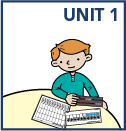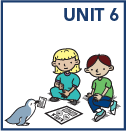top of page

# Plan de estudios en MRA

• Solve addition and subtraction story problems

• Count by ones and tens to 120 read and write numbers to 120, and represent a number of objects up to 120 with a written numeral

• Understand place value by describing what the digits mean in two-digit numbers; use this knowledge to add and subtract

• Add two-digit numbers using at least two different strategies and explain how the strategies work

• Subtract two-digit numbers that are multiples of 10 using at least two different strategies and explain how the strategies work

• Compare two numbers using the symbols >, =, and <

• Add and subtract numbers within 10 efficiently and accurately

• Measure length using non-standard units such as Popsicle sticks, linking cubes, and so on

• Tell and write time to the hour and half-hour on analog and digital clockbottom of page